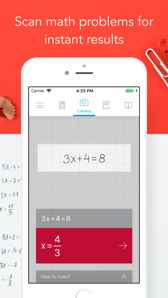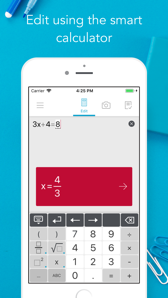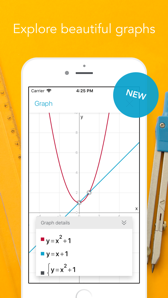Photomath

iPhoneAPP ID

919087726

4.2.3

••••Simply point your camera toward a math problem and Photomath will magically show the result with a detailed step-by-step instructions.

Photomath provides:

• Camera calculator

• Handwriting recognition

• Step-by-step instructions

• Smart calculator

• Graphs (NEW)

Photomath supports arithmetics, integers, fractions, decimal numbers, roots, algebraic expressions, linear equations/inequalities, quadratic equations/inequalities, absolute equations/inequalities, systems of equations, logarithms, trigonometry, exponential and logarithmic functions, derivatives and integrals.

• Crash fix

• Improved solution steps and step explanations

• Various fixes and improvements to math problem recognition

• Various UI fixes and performance improvements

We bring updates regularly to make math learning and problem solving even easier for you! All new major features will be introduced inside the app.

• 开发商：Photomath, Inc.
• 类别：Education